Next: GVF and Shape Analysis Up: Gray-level Images and Higher Previous: Gray-level Images

## Higher Dimensions

GVF can be easily generalized to higher dimensions. Let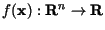be an edge map defined in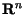. The GVF field inis defined as the vector field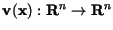which minimizes the energy functional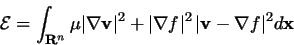(14)

where the gradient operator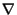is applied to each component of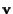separately. Using the calculus of variations, we find that the GVF field must satisfy the Euler equation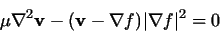(15)

where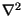is also applied to each component of the vector fieldseparately.

A solution to these Euler equations can be found by introducing a time variable t and finding the steady-state solution of the following linear parabolic partial differential equation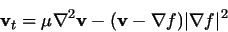(16)

where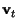denotes the partial derivative ofwith respect to t. Equation (20) comprises n decoupled scalar linear second order parabolic partial differential equations in each element of. Therefore, in principle, it can be solved in parallel. In analogous fashion to the 2-D case, finite differences can be used to approximate the required derivatives and each scalar equation can be solved iteratively.

A preliminary experiment using GVF in three dimensions was carried out using the object shown in Fig. 7a. This shape was created on a 643 grid, and rendered using an isosurface algorithm. The roughness of its surface reflects the relative coarseness of the grid, although the isosurface algorithm makes it somewhat smoother because of its ability for sub-pixel resolution. We computed the 3-D GVF field for this data set using a numerical approximation to (20) and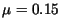. This result on the two planes shown in Fig. 7b, is shown projected onto these planes in Figs. 7c and d. The same characteristic properties that we observed about GVF in 2-D are apparent here as well.

Next, a deformable surface  was initialized as the sphere shown in Fig. 7e; it is neither entirely inside nor entirely outside the object. The surface was then allowed to move in response to the GVF external forces and its own internal forces. Intermediate results after 10 and 40 iterations are shown in Figs. 7f and g. The final result after 100 iterations is shown in Fig. 7h. It is interesting to note that the final active surface is much smoother than the isosurface rendering. This is because the internal forces of the active surface favor smoother surfaces.Next: GVF and Shape Analysis Up: Gray-level Images and Higher Previous: Gray-level Images
Chenyang Xu
1999-11-06